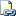# NTILE (Transact-SQL)

Distributes the rows in an ordered partition into a specified number of groups. The groups are numbered, starting at one. For each row, NTILE returns the number of the group to which the row belongs.Transact-SQL Syntax Conventions

## Syntax

``````NTILE (integer_expression)    OVER ( [ <partition_by_clause> ] < order_by_clause > )
``````

## Arguments

• integer_expression
Is a positive integer constant expression that specifies the number of groups into which each partition must be divided. integer_expression can be of type int, or bigint.

Note

integer_expression can only reference columns in the PARTITION BY clause. integer_expression cannot reference columns listed in the current FROM clause.

• <partition_by_clause>
Divides the result set produced by the FROM clause into partitions to which the RANK function is applied. For the syntax of PARTITION BY, see OVER Clause (Transact-SQL).
• < order_by_clause >
Determines the order in which the NTILE values are assigned to the rows in a partition. For more information, see ORDER BY Clause (Transact-SQL). An integer cannot represent a column when the <order_by_clause> is used in a ranking function.

bigint

## Remarks

If the number of rows in a partition is not divisible by integer_expression, this will cause groups of two sizes that differ by one member. Larger groups come before smaller groups in the order specified by the OVER clause. For example if the total number of rows is 53 and the number of groups is five, the first three groups will have 11 rows and the two remaining groups will have 10 rows each. If on the other hand the total number of rows is divisible by the number of groups, the rows will be evenly distributed among the groups. For example, if the total number of rows is 50, and there are five groups, each bucket will contain 10 rows.

## Examples

### A. Dividing rows into groups

The following example divides the rows into four groups. Because the total number of rows is not divisible by the number of groups, the first group has four rows and the remaining groups have three rows each.

``````USE AdventureWorks;
GO
SELECT c.FirstName, c.LastName
,NTILE(4) OVER(ORDER BY SalesYTD DESC) AS 'Quartile'
,s.SalesYTD, a.PostalCode
FROM Sales.SalesPerson s
INNER JOIN Person.Contact c
ON s.SalesPersonID = c.ContactID
WHERE TerritoryID IS NOT NULL
AND SalesYTD <> 0;
GO
``````

### B. Dividing the result set by using PARTITION BY

The following example adds the `PARTITION BY` argument to the code in example A. The rows are first partitioned by `PostalCode` and then divided into four groups within each `PostalCode`. Notice that the `ORDER BY` in the `OVER` clause orders the `NTILE` and the `ORDER BY` of the `SELECT` statement orders the result set.

``````USE AdventureWorks;
GO
SELECT c.FirstName, c.LastName
,NTILE(4) OVER(PARTITION BY PostalCode ORDER BY SalesYTD DESC) AS 'Quartile'
,s.SalesYTD, a.PostalCode
FROM Sales.SalesPerson s
INNER JOIN Person.Contact c
ON s.SalesPersonID = c.ContactID